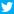「文化工房」による調査データ一覧 | 調査のチカラ

他にはこんな会社も

胸キュン映画がズラリ…10代が選ぶ最も好きな青春映画

').f(b.get(["line2"],!1),b,"h").f(b.get(["line3"],!1),b,"h").w(' ').f(b.get(["visible_url"],!1),b,"h").w("").h("ne",b,{"else":f,block:k},{key:b.get(["toSellText"],!1),value:""},"h").w("").h("ne",b,{"else":f,block:k},{key:b.get(["toSellText"],!1),valu

トップはあの不朽の名作…30代が選ぶ最も好きな青春映画

トップはあの不朽の名作…30代が選ぶ最も好きな青春映画

2017年3月27日文化工房フィードバック数：0マナーを注意したら逆ギレ…20代が旅行でした一番の失敗

').f(b.get(["line2"],!1),b,"h").f(b.get(["line3"],!1),b,"h").w(' ').f(b.get(["visible_url"],!1),b,"h").w("").h("ne",b,{"else":f,block:k},{key:b.get(["toSellText"],!1),value:""},"h").w("").h("ne",b,{"else":f,block:k},{key:b.get(["toSellText"],!1),valu

使い方は現実的? 50代が最も欲しいドラえもんの道具

2017年3月11日文化工房フィードバック数：0子どもの将来が心配…50代が思うバカヤローな相手

').f(b.get(["line2"],!1),b,"h").f(b.get(["line3"],!1),b,"h").w(' ').f(b.get(["visible_url"],!1),b,"h").w("").h("ne",b,{"else":f,block:k},{key:b.get(["toSellText"],!1),value:""},"h").w("").h("ne",b,{"else":f,block:k},{key:b.get(["toSellText"],!1),valu

ママ友、ご近所、店員も…バカヤローな相手 少数派

').f(b.get(["line2"],!1),b,"h").f(b.get(["line3"],!1),b,"h").w(' ').f(b.get(["visible_url"],!1),b,"h").w("").h("ne",b,{"else":f,block:k},{key:b.get(["toSellText"],!1),value:""},"h").w("").h("ne",b,{"else":f,block:k},{key:b.get(["toSellText"],!1),valu

男女で唯一共通する人気者は? 10代が好きな猫のキャラ

').f(b.get(["line2"],!1),b,"h").f(b.get(["line3"],!1),b,"h").w(' ').f(b.get(["visible_url"],!1),b,"h").w("").h("ne",b,{"else":f,block:k},{key:b.get(["toSellText"],!1),value:""},"h").w("").h("ne",b,{"else":f,block:k},{key:b.get(["toSellText"],!1),valu

“猫感”はジジに軍配、30代が最も好きな猫のキャラ

2017年2月25日文化工房フィードバック数：0キティにジブリ作品も! 好きな猫キャラTOP5、1位は?

').f(b.get(["line2"],!1),b,"h").f(b.get(["line3"],!1),b,"h").w(' ').f(b.get(["visible_url"],!1),b,"h").w("").h("ne",b,{"else":f,block:k},{key:b.get(["toSellText"],!1),value:""},"h").w("").h("ne",b,{"else":f,block:k},{key:b.get(["toSellText"],!1),valu

ピンクは可愛くて実用的、20代が好きなブラジャーの色

ピンクは可愛くて実用的、20代が好きなブラジャーの色

女性が選ぶ“オトナ色”…50代が一番好きなブラジャーの色

').f(b.get(["line2"],!1),b,"h").f(b.get(["line3"],!1),b,"h").w(' ').f(b.get(["visible_url"],!1),b,"h").w("").h("ne",b,{"else":f,block:k},{key:b.get(["toSellText"],!1),value:""},"h").w("").h("ne",b,{"else":f,block:k},{key:b.get(["toSellText"],!1),valu

このページをシェア

「調査のチカラ」は無料で閲覧できる、たくさんの調査データのリンク集です。検索機能も備えていますので、調査データが他のどこよりも簡単に探せます。
インターネットの発展によって、多くの企業が貴重なデータをオンラインに公開してくれるようになりました。それらの”調査のチカラ”を皆さんに活用していただくことで、データを公開してくれている企業にももっと光が当たるようになればうれしいです。
※ご紹介しているデータの利用許諾や購入については提供元ごとに対応が異なります。是非とも各リンク先にてご相談ください。
もう少し詳しい自己紹介ページはこちらRSSFacebookTwitterメルマガ

日付で探す

2019年6月
1
2 3 4 5 6 7 8
9 10 11 12 13 14 15
16 17 18 19 20 21 22
23 24 25 26 27 28 29
30